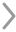WHCSRL 技术网

# 4万字【Python进阶编程】保姆式教学，觉得进阶难？学完这些就简单了## ➿（一）面向对象的思想## ➿（二）面向对象的两个重要概念：类和对象

#### 1.对象和类的概念#### 2.对象详解

（1）对象的组成： 对象=属性+方法

（2）对象的属性有哪些特征

• 对象的属性可以是抽象的属性

• 对象的属性可以是另一个对象

• 大对象由小对象所组成（3）对象的方法

#### 3.类的详解

（1）创建类

``````class 类名：
属性1
属性2
方法1
方法2
#1个类中可以有多个属性和方法，也可以只有属性或者只有方法
```123456```

``````#创建Student类
class Student:
name = "小明"	#学生类的name属性
age = 18	#学生类的age属性
def learn(self):	#学生类的learn方法
print("学生的学习方法被调用了")

#创建Student类的对象
s1 = Student() #Student()就是创建的1个类对象，只是便于简写，我把它传给了s1
s1.learn()	#对象的方法
print(s1.age)	#执行对象的属性

#上面这两行代码其实可以直接写成Student().learn() ，也同样是利用类创建了对象并调用对象的方法
```12345678910111213```

``````Student().learn()	#创类的对象并使用对象的方法
print(Student().age)	#创建类的对象并执行对象的属性
```12```#### （1）属性详解

• 类属性
``````1. 类属性定义在类的内部，任何方法之外
1. 创建类的时候直接创建
2. 类名.新的属性名=属性值
2. 使用类属性：
1. 对象.类属性
2. 类.类属性
3. 类属性的特点：
1. 类属性是属于类对象的
2. 对象共用类属性。如果类属性变化，所有的对象也会改变
4. 类属性有且只有一份，牵一发而动全局
```12345678910```
• 对象属性
``````对象属性定义在方法内部
1. 创建
1. 对象.实例属性名=属性值
2. 方法内部创建：self.属性名 = 属性值
2. 使用对象属性
对象.实例属性名
3. 对象属性各自拥有互相独立
4. 对象属性和类属性发生命名冲突
1. 以对象属性名优先
2. 对象属性名会遮蔽同名的类属性
```12345678910```

#### （2）类的方法详解

• 类方法的声明
``````def 函数名（self,参数1，参数2，....）
```1```
• self
``````1.self默认传递，不需要传值
2.self指代的是当前的实例（对象）
3.一定会有self传递，但名字不一定必须叫self，可以叫aa
4.self后面可以跟多个参数，用“，”隔开
```1234```
• 方法的调用
``````1.对象.方法名（自动传递对象），例如 s2.fun1()
2.类名.方法名（类名（）） #“类名（）”相当于创建对象

```1234```
• 初始化的方法（特殊的类的方法）
``````def __init__():

1.这个方法不需要被调用，只要创建对象就会自动执行
2.这个方法只能返回None值，无法返回其他类型的值
3.如果要给init传递多个参数，只能通过 类名（多个参数） 的方式去传递
4.如果类里面没有定义init，默认去调用父类
5.如果类里面重复定义了多个init方法，会被最后一个init方法覆盖
```1234567```

``````#创建类
class Student:
name = "小莫"
age  = "18"
def __init__(self,aa,bb):
print("init被调用了")
print(aa)
print(bb)
def fun1(self):
print("函数1")
print(self)
def fun2(self):
print("函数2")

Student.fun1(Student(100,200)) #创建对象并传递多个值给init
```123456789101112131415```

``````init被调用了
100
200

<__main__.Student object at 0x000001DAD8168400>	#self值的存储地址
```12345```

## ➿（三）面向对象的三大特性

#### 1.封装

1.对象都有明确的边界，把属性和方法保护在边界之内。（安全性）

2.封装的力度适中。

3.封装的原则

``````（1）将不需要对外提供的内容进行隐藏。
（2）隐藏属性，提供公共的方法对其访问
私有属性：__name="xxx"
```123```

#### 2.继承

（1）定义形式（类的完整形式）

``````	class 子类的类名（父类的类名）：
属性
方法
```123```

（2）父类：基类，超类

``````	object————顶层类
如果对象没有书写继承关系，默认继承object
```12```

（3）继承的特点

• 子类可以继续父类的属性和方法

``````# 定义父类
class A:
name1 = "父类的属性1"

def aa(self):
print("父类的方法1")

# 定义子类
class B(A):
name2 = "子类的属性1"

def bb(self):
print("子类的方法1")

n = B()
print(n.name2)	#调用子类的属性
n.bb()	#调用子类的方法
print(n.name1)	#调用父类的属性
n.aa()	#调用父类的方法

```1234567891011121314151617181920```

``````子类的属性1

```1234```
• 可扩展性。父类扩展了，子类也得到扩展。

• 如果子类没有构造方法，对象会去调用父类的。

• 如果子类有自己的构造方法，则不会去调用父类的。

• 子类的构造方法可以调用父类的构造方法，调用可以有以下两种方式：

``````父类的类名.__init__(self)	#手动传递self
super().__init__()	#不需要加self
```12```
• 多继承

``````# 定义父类A
class A:
name_a = "父类A的属性1"
def aa(self):
print("父类A的方法1")

# 定义父类B
class B:
name_b = "父类B的属性1"
def bb(self):
print("父类B的方法1")

#定义子类C
class C(A,B): #继承两个父类
pass #跳过

n = C()
print(n.name_a)
print(n.name_b)
n.aa()
n.bb()
```123456789101112131415161718192021```

``````父类A的属性1

```1234```

（4）方法覆盖

• 子类中的方法与父类中的方法同名，则覆盖父类。
``````#定义父类
class Animal:
def eat(self):
print("动物会吃东西")

#定义子类狗
class dog(Animal):
def eat(self):
print("狗会吃东西")

d = dog()
d.eat()
```123456789101112```

``````狗会吃东西
```1```
• 子类覆盖了覆盖的方法之后，本质上并没有替换父类的方法，父类的方法依然存在并可以给其他子类调用。

• 方法覆盖的前提：子类的方法名必须和父类的完全相同

（5）方法重载#### 3.多态和多态性

``````#定义父类Hero
class Hero :
def stroke(self):   #技能函数
print("英雄技能")

#定义子类程咬金
class ChengYaoJin(Hero):
def stroke(self):   #技能函数
print("一技能跳跃，二技能旋转，大招回血")

#定义子类后裔
class HouYi(Hero):
def stroke(self):   #技能函数
print("一技能多发，二技能射圈，大招大鸟眩晕")

#定义调用者
class Person:
def chose_hero(self,hero):  #玩家选择英雄
hero.stroke()

c = ChengYaoJin()   #程咬金
x = HouYi()     #后裔
p = Person()    #玩家
p.chose_hero(c)     #玩家选择程咬金，"c"换成"x"就会产生不同的结果
```123456789101112131415161718192021222324```

``````一技能跳跃，二技能旋转，大招回血
```1```

``````#定义鸟类
class Bird:
def fly(self):
print("鸟会飞")

#定义飞机类
class Plane:
def fly(self):
print("飞机会飞")

#定义火箭类
class Rocket:
def fly(self):
print("火箭会飞")

#函数调用父类的fly方法
def fun1(obj):
obj.fly()

fun1(Bird())    #调用鸟类的fly方法
fun1(Plane())   #调用飞机类的fly方法
fun1(Rocket())  #调用火箭类的fly方法
```12345678910111213141516171819202122```

``````鸟会飞

```123```

## 👳（一）模块

#### 2.模块导入

Python中允许导入的形式来进行模块调用，Python中的模块也是对象，模块中定义的所有全局变量，导入后都成为模块的属性。

``````import math
num = math.sqrt(9)
print(num)
```123```

``````3.0
```1```

``````from math import sqrt
num = sqrt(9)
print(num)
```123```

``````3.0
```1```

``````这个方式并非在所有情况下都能导入模块中的所有功能，

```1234```

``````def fun1() : #实现a+b并输出结果
print("fun1")

def fun2() : #实现a+b并输出结果
print("fun2")
__all__ = ["fun1"]  #声明只能调用fun1()
```123456```

``````from module1 import *
fun1()
fun2()
```123```

``````在module1中用__all__声明了可调用的方法只有fun1()。
```1```

#### 3.定义别名

1.模块自定义别名： import 模块名 as 别名

``````import time as t
t.sleep(3)  #延迟三秒
print("hello world")
```123```

``````hello world
```1```

2.功能自定义别名： from 模块名 import 功能名 as 别名

``````from time import sleep as s
s(5)	#延时5秒
print("hello world")
```123```

``````hello world
```1```

#### 4.自定义模块

``````def fun1(a,b) : #实现a+b并输出结果
print(a+b)
```12```

``````import module1
module1.fun1(20,30)
```12```

``````50
```1```

#### 5.模块的测试

``````很多模块在内部都有测试方法：
if __name__ == "__main__":
代码1
```123```

``````print(__name__) #打印__name__
```1```

``````__main__
```1```

``````import module1
```1```

``````module1
```1```

## 👳（二）包

#### 1.定义

``````包就是将有联系的模块放在同一个文件夹下，并且该文件夹里有“__init__.py”这个文件，这个文件夹就叫做包。
```1```

``````1.包也是对象
2.必须有__init__.py文件
3.__init__.py文件是包的构造方法，控制着包的导入行为，一般是个空包
4.包里面不仅可以有模块，还可以有子包
```1234```

#### 2.包的创建#### 4.包的使用

``````import 包名.模块名

```12```

``````a = 100
def fun1():
print("这是1个包")
```123```

``````import  demo.my_module
print(demo.my_module.a)
demo.my_module.fun1()
```123```

``````100

```12```

``````from 包名 import 模块名

```123```

``````from demo import my_module
print(my_module.a)
my_module.fun1()
```123```

``````100

```12```

``````import 包名.模块名 as 别名

```123```

``````import  demo.my_module as n1    #另起别名为n1
print(n1.a)
n1.fun1()
```123```

``````100

```12```

``````from 包名.模块名 import 功能1

```12```

``````from demo.my_module import fun1
fun1()
```12```

``````这是1个包
```1```

``````def fun1():
print("这是功能1")
def fun2():
print("这是功能2")
```1234```

``````from demo.hhhh import *
fun1()
fun2()
```123```

``````这是功能1

```12```

6.关于all的使用

``````__all__ = [
"hhhh"  # hhhh模块允许被导入
]
```123```

``````from demo import *	#导入包里面的所有模块
hhhh.fun1()		#调用hhhh模块的fun1功能，输出“这是功能1”
my_module.fun1()	#调用my_module模块的fun1功能，输出“这是1个包”
```123```## 👳（三）模块与包的作用

1.提高代码的可重用性。好用的代码不止你1个人可以用，很多人都可以重复使用它。

2.提高代码的可读性。如果所有的代码都放在1个.py文件中，那代码就太长了，增加了理解和维护难度，所以可以把一些常用的代码封装成包和模块，起1个望文生义的名字，需要的时候直接用就行，减少了代码的数量，提高了可读性。

3.减少代码的冗余。模块里面封装的一些方法，我们直接给参数去使用就可以了，不用把方法再写一遍，占用内存，也就减少了代码的冗余。

## 👳（四）第三方库的安装

Python虽然有很多自带的模块和包，简称内置模块，但只会使用内置模块还不够，毕竟内置模块有限，我们经常会用到第三方的库，这个时候学习怎么安装第三方的库（包）是很必要的。

#### 1.通过pip安装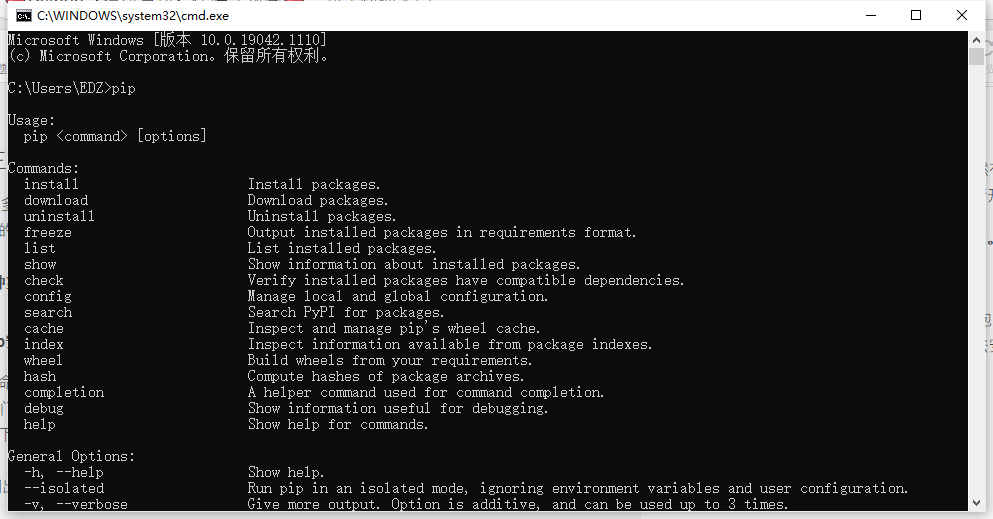``````1.WIN+R调出运行窗口
2.输入cmd
3.输入 pip install Pillow
4.等待下载和安装的完成
```1234```（1）.pip版本过低，升级一下pip版本，在黑窗口输入：python -m pip install -U pip

（2）网络不好，多下载几遍就可以了

#### 2.通过Pycharm安装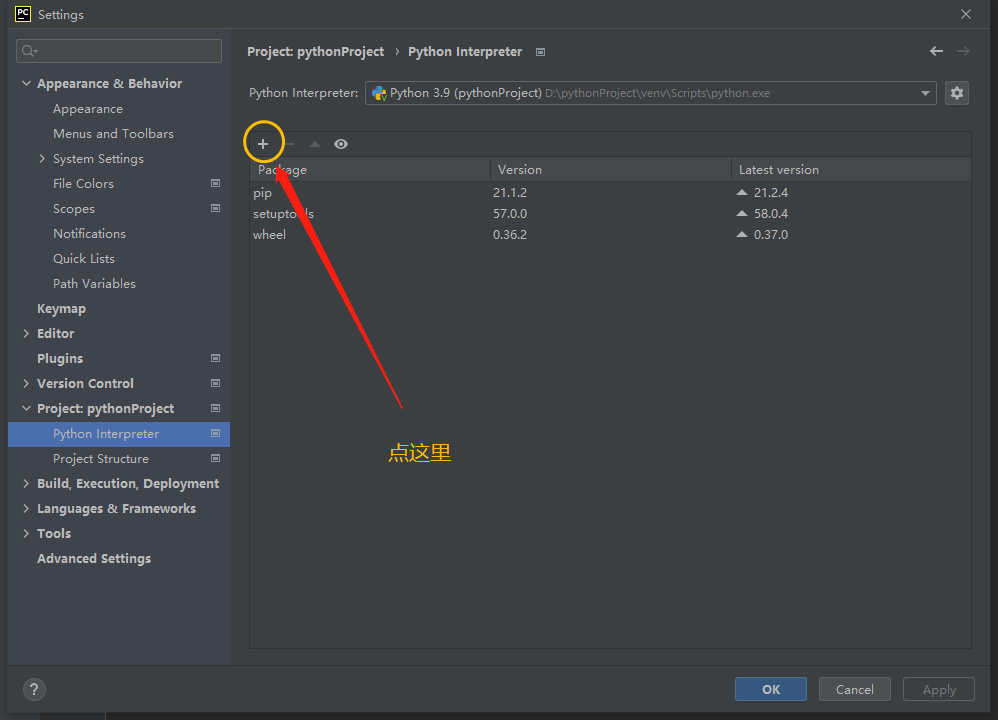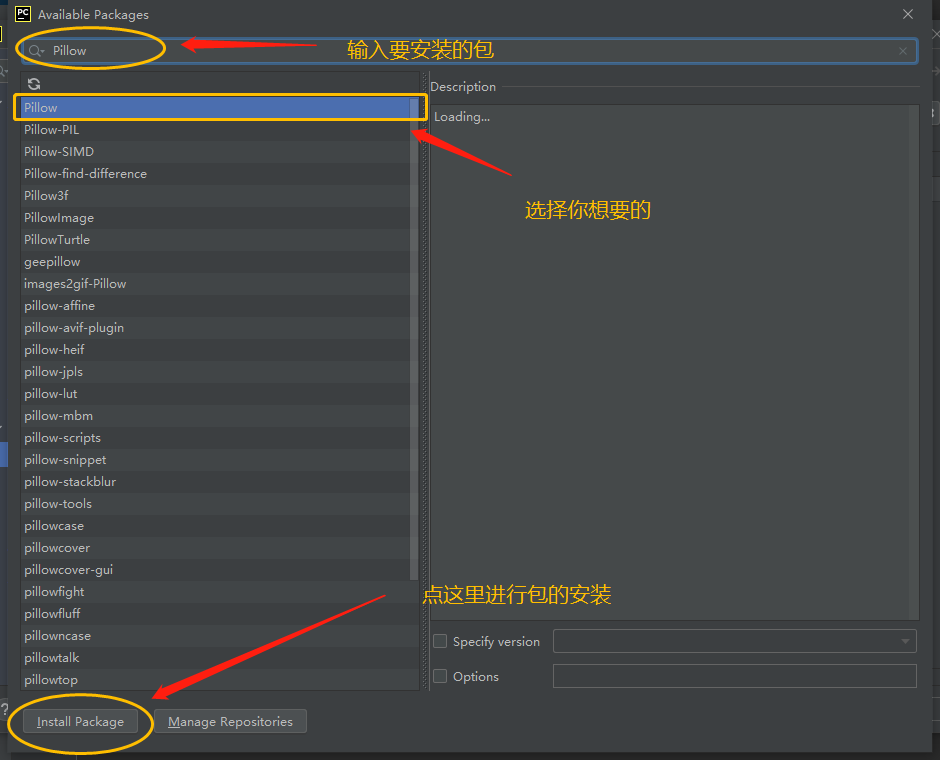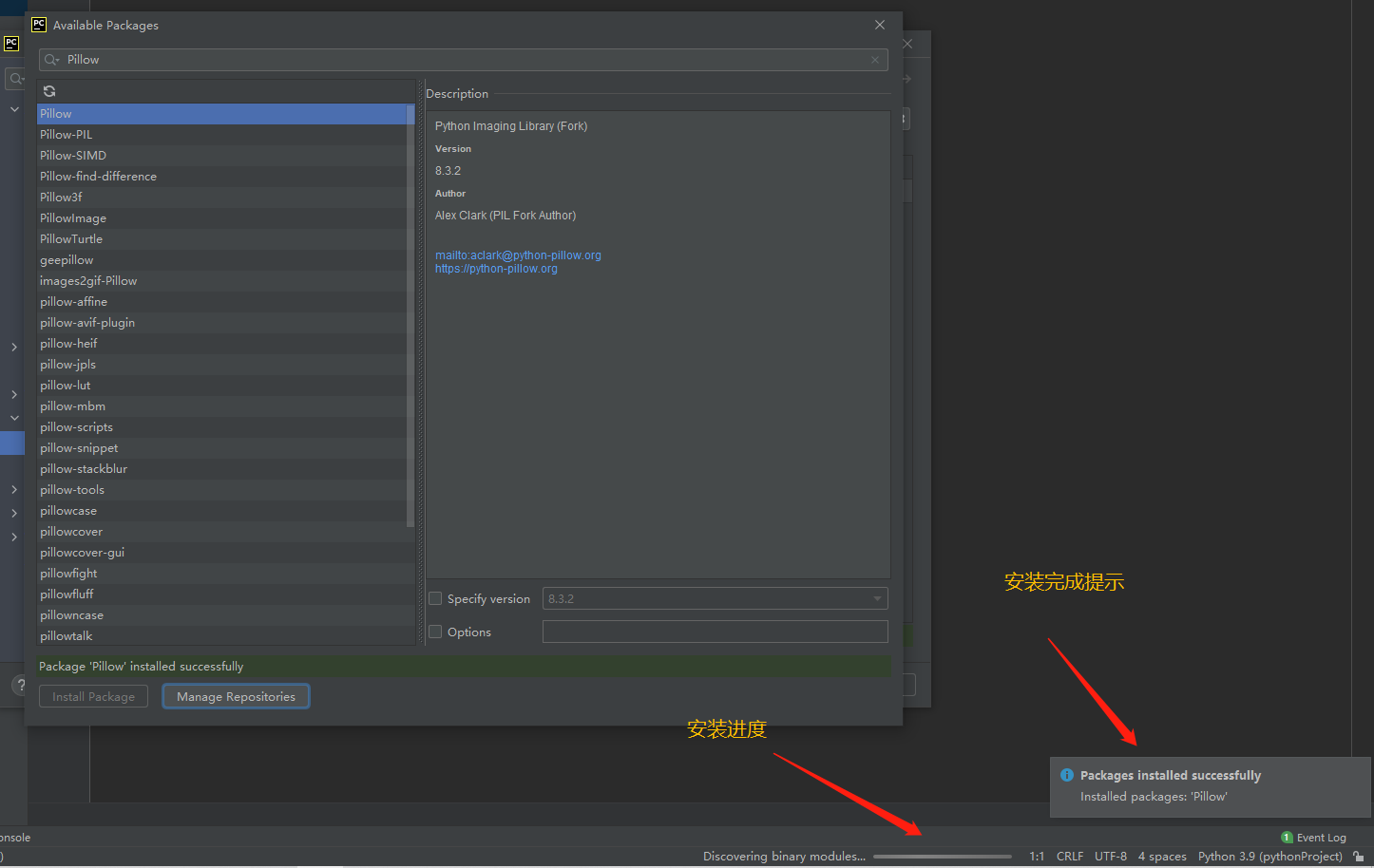#### 3.通过渠道下载.whl文件安装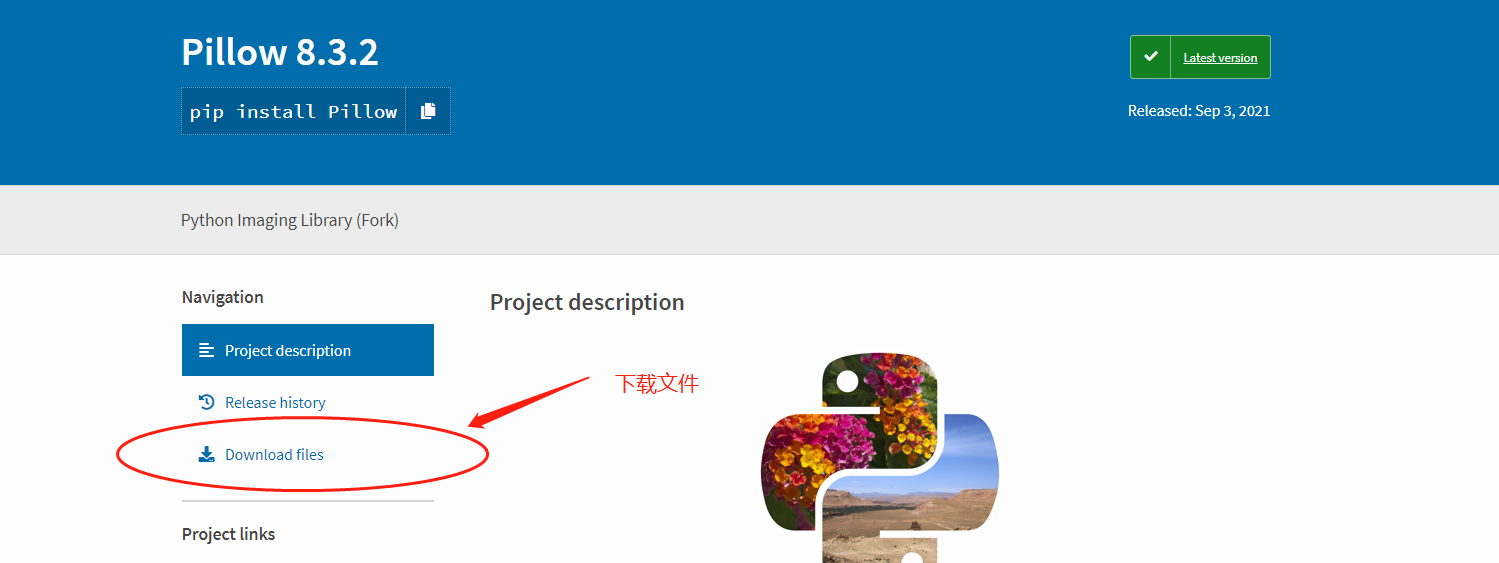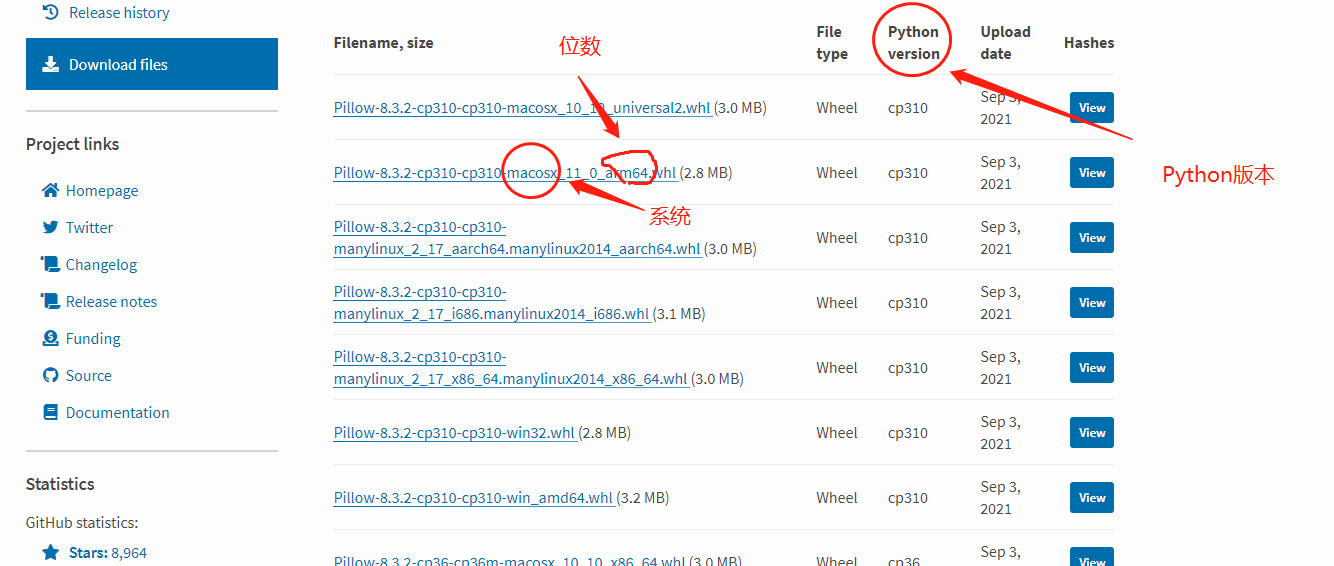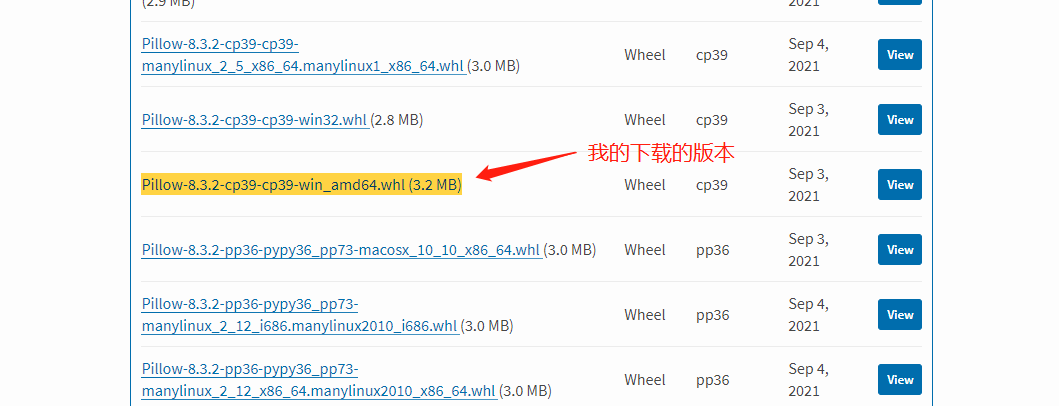``````pip install D:谷歌浏览器Pillow-8.3.2-cp39-cp39-win_amd64.whl
```1```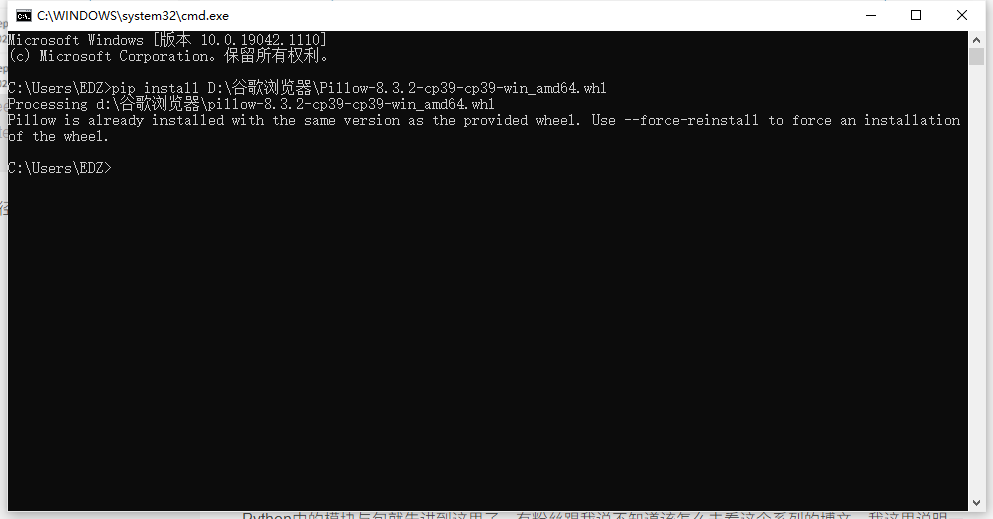## 💼（一）文件的定义和操作## 💼（二）文件的基本操作

1.open(name,mode) ----打开文件

name指的是文件名，一定要写全，何为写全？就是要写清楚 存储路径+文件名+后缀 。

mode是打开文件的模式，默认是r，也就是只读的方式。mode的方式有很多，比如读、写等等，后面我们会讲到。

2.write(“内容") ------写

4.close() ------关闭文件

5.案例

``````f = open("文件一.txt",'w') #以写入的方式，打开文件
f.write("hello world")  #向文件一中写入内容
f.close()   #关闭文件
```123`````````f = open("文件一.txt",'r') #以写入的方式，打开文件
f.close()   #关闭文件
```123```

``````hello world
```1```

``````f = open("文件一.txt",'r')	#只读的方式打开文件
```1```

6.mode的其他操作模式

mode中有很多的操作模式，我们以表格的方式来看看：

r以只读的形式打开文件，文件的指针在开头
r+读写，文件指针在开头
rb以二进制的形式，只读文件指针在开头
w只写，文件不存在，则创建新的，存在则覆盖，指针在开头
w+读写，文件不存在，则创建新的，存在则覆盖，指针在开头
wb只写，以二进制的形式
a追加模式，文件指针在结尾
a+读写，不存在则创建，存在直接追加
ab以二进制形式追加

``````f = open("test.txt", 'a+')
f.write("aaa")
f.close()

f = open("test.txt")  # 默认只读模式

f = open("test.txt", 'a+')
f.write("bbb")
f.close()

f = open("test.txt")  # 默认只读模式

```1234567891011121314```

``````aaa
aaabbb
```12```

## 💼（三）文件的方法与属性

1.file的对象属性

``````1. closed
如果文件对象已关闭，返回True，否则返回False
2. mode
返回文件对象的访问模式
3. name
返回文件的名称
```123456```

``````f = open("test.txt", 'a+')
f.write("aaa")
f.close()

print(f.closed) #查看是否关闭
print(f.name)   #查看文件名字
print(f.mode)   #查看操作模式
```1234567```

``````True
test.txt
a+
```123```

2.file的对象方法

``````1. close()
关闭文件---非常重要
读取文件中的内容
count：字节数量
读取所有内容，打包成列表
读取一行数据，追加读取，读取过的不能再次读取
5. seek（offset，[from]）
修改指针的位置：从from位置移动了offset个字节
from：0则表示从起始位置，1则表示从当前位置开始，2则表示从末尾开始
oofset：要移动的字节数
6. write()
向文件中写入内容
```123456789101112131415```

``````f = open("test.txt", 'a+')
f.write("aaabbbccc")
f.close()

f = open("test.txt")
f.close()
```1234567```

``````['aaabbbcccaaabbbccc']
```1```

## 💼（四）os模块

os模块是一个用于访问操作系统的模块，在进行文件操作的时候常会用到它。模块在使用之前要进行导入。

``````import os
```1```

1.关于文件的功能

``````1.os.rename(原文件名，新的文件名)	——文件重命名
2.os.remove(文件名)	——删除文件
如果不说明路径，则在源代码所在文件夹下寻找，寻找不到会报错。
若想删除指定文件夹下的文件，文件名则需要具体路径，例如 os.remove(r"D:	est_1文件名"),r防止斜杠发生转义
```1234```

``````import os
os.rename("test1.txt","test20.txt")
```12```2.文件夹的功能

``````1.os.mkdir(文件夹名)	——创建文件夹
2.os.rmdir(文件夹名)	——删除文件夹
3.os.getced()	——获取当前目录
4.os.chdir(目录)	——切换目录
5.os.listdir(目录)	——获取当前文件夹下所有的文件或者文件夹，返回一个列表
os.listdir("aa") #获取aa文件夹下的所有文件或文件夹，返回一个列表
```123456```

``````import os

os.chdir(r"D:文件venv")  #切换到venv文件夹下,r是防止转义
os.mkdir("新建文件夹")   #在venv文件夹下创建一个新建文件夹
print(os.getcwd())  #输出当前目录位置
```12345```## ⚡（一）异常的定义## ⚡（二）异常的处理

#### 1.try-except

``````try:
有可能出现异常的代码
except 异常类型 as 变量
处理后的代码
```1234`````````try:
print(a)
except NameError as s:
print(s)
```1234```#### 2.try-except-except

``````try:
1 / 0
print(a)
except NameError as s:  # 第一种写法，用as+变量
print(s)
except ZeroDivisionError:  # 第二种写法，自定义输出内容
print("除数不能为0")  # 自定义输出的内容
```1234567```try-except的写法很灵活的，我们同样可以用元组把可能报错的异常类型囊括进去，避免写多行except，例如：#### 3.try-except-else#### 4.try-except-finally#### 5.顶层类Exception

except后面其实可以不加错误类型，因为系统会默认认为后面的错误是类型是Exception，这是1个顶层类，包含了所有的出错类型。## ⚡（三）自定义异常

``````class MyError(Exception):  # 异常捕获的类
def __init__(self, length, min_len):  # length为用户输入的密码长度，min_len为规定的最小长度
self.length = length
self.min_len = min_len

# 设置抛出异常的描述信息
def __str__(self):
return "你输入的长度是%%%%%%%%%%%%%%%%%%%%%%%%%%%%%%%%%%%%%%%%%%%%%%%%%%%%%%%%%%%%%%%%s,不能少于%%%%%%%%%%%%%%%%%%%%%%%%%%%%%%%%%%%%%%%%%%%%%%%%%%%%%%%%%%%%%%%%s" %%%%%%%%%%%%%%%%%%%%%%%%%%%%%%%%%%%%%%%%%%%%%%%%%%%%%%%%%%%%%%%% (self.length, self.min_len)

def main():
try:
con = input("请输入密码：")  # 获取用户输入的密码
l = len(con)  # 获取用户输入的密码长度
if l < 6:
raise MyError(l, 6)  # 长度低于设定的6位数则引发异常
except Exception as ss:  # 有错误则提示
print(ss)
else:
print("您的密码输入完毕")  # 没有错误则执行

main()
```1234567891011121314151617181920212223```## ✈️（一）re模块

1.导入re模块

``````import re
```1```

2.findall（）的语法：

``````findall(正则表达式，目标字符串)
```1```

## ✈️（二）正则表达式

#### 1.字符串的匹配

（1）普通字符

``````import re
a = "abc123+-*"
b = re.findall('abc',a)
print(b)
```1234```

``````['abc']
```1```

（2）元字符** 1️⃣ []**

[] 的使用方式主要有以下三种：

• 常用来指定一个字符集。
``````s = "a123456b"
rule = "a[0-9][1-6][1-6][1-6][1-6][1-6]b"	#这里暂时先用这种麻烦点的方法，后面有更容易的，不用敲这么多[1-6]
l = re.findall(rule,s)
print(l)
```1234```

``````['a123456b']
```1```
• 可以表示一个范围。

``````s = "abcabcaccaac"
rule = "a[a,b,c]c"  # rule = "a[a-z0-9][a-z0-9][a-z0-9][a-z0-9]c"
l = re.findall(rule, s)
print(l)
```1234```

``````['abc', 'abc', 'acc', 'aac']
```1```
• [] 内的元字符不起作用，只表示普通字符。

``````print(re.findall("caa[a，^]", "caa^bcabcaabc"))
```1```

``````['caa^']
```1```

``````print(re.findall("caa[^,a]", "caa^bcabcaabc"))
```1```

``````['caa^', 'caab']
```1```

2️⃣^

^ 通常用来匹配行首，例如：

``````print(re.findall("^abca", "abcabcabc"))
```1```

``````['abca']
```1```

3️⃣ \$
\$ 通常用来匹配行尾，例如：

``````print(re.findall("abc\$", "accabcabc"))
```1```

``````['abc']
```1```

4️⃣

​ 反斜杠后面可以加不同的字符表示不同的特殊含义，常见的有以下3种。

• d:匹配任何十进制数等价于[0-9]
``````print(re.findall("cddda", "abc123abc"))
```1```

``````['c123a']
```1```

``````print(re.findall("^abc", "^abc^abc"))
```1```

``````['^abc', '^abc']
```1```

5️⃣ s

``````print(re.findall("ss", "a     c"))
```1```

``````['  ', '  ']
```1```

6️⃣ w

``````print(re.findall("www", "abc12_"))
```1```

``````['abc', '12_']
```1```

7️⃣ {n}

{n}可以避免重复写，比如前面我们用w时写了3次w，而这里我们这需要用用上{n}就可以，n表示匹配的次数，例如：

``````print(re.findall("w{2}", "abc12_"))
```1```

``````['ab', 'c1', '2_']
```1```

8️⃣ *

*表示匹配零次或多次（尽可能的多去匹配），例如：

``````print(re.findall("010-d*", "010-123456789"))
```1```

``````['010-123456789']
```1```

9️⃣ +

+表示匹配一次或多次，例如

``````print(re.findall("010-d+", "010-123456789"))
```1```

``````['010-123456789']
```1```

🔟 .

.是个点，这里不是很明显，它用来操作除了换行符以外的任何字符，例如：

``````print(re.findall(".", "010
?!"))
```1```

``````['0', '1', '0', '?', '!']
```1```

1️⃣ 1️⃣ ？

？表示匹配一次或零次

``````print(re.findall("010-d?", "010-123456789"))
```1```

``````['010-1']
```1```

``````print(re.findall("010-d*", "010-123456789"))
```1```

``````['010-123456789']
```1```

``````print(re.findall("010-d*?", "010-123456789"))
```1```

``````['010-']
```1```

1️⃣2️⃣{m,n}
m,n指的是十进制数,表示最少重复m次，最多重复n次，例如：

``````print(re.findall("010-d{3,5}", "010-123456789"))
```1```

``````['010-12345']
```1```

``````print(re.findall("010-d{3,5}?", "010-123456789"))
```1```

``````['010-123']
```1```

{m,n}还有其他的一些灵活的写法，比如：

• {1,} 相当于前面提过的 + 的效果
• {0，1} 相当于前面提过的 ？ 的效果
• {0，} 相当于前面提过的 * 的效果

## ✈️（三）正则的使用

#### 1.编译正则

`````` s = "010-123456789"
rule = "010-d*"
rule_compile = re.compile(rule) #返回一个对象
# print(rule_compile)
s_compile = rule_compile.findall(s)
print(s_compile)	#打印compile()返回的对象是什么
```123456```

``````['010-123456789']
```1```

#### 2.正则对象的使用方法

（1）findall()

（2）search()

（3）match()

``````s = "010-123456789"
rule = "010-d*"
rule_compile = re.compile(rule)  # 返回一个对象
# print(rule_compile)
s_compile = rule_compile.match(s)
print(s_compile)  # 打印compile()返回的对象是什么
```123456```

``````<re.Match object; span=(0, 13), match='010-123456789'>
```1```

#### 3.Match object 的操作方法

（1）group()

（2）start()

（3）end()

（4）span()

``````s = "010-123456789"
rule = "010-d*"
rule_compile = re.compile(rule)  # 返回一个对象
s_compile = rule_compile.match(s)
print(s_compile.span())  #用span()处理返回的对象
```12345```

``````(0, 13)
```1```

#### 4.re模块的函数

re模块中除了上面介绍的findall()函数之外，还有其他的函数，来做一个介绍：

（1）findall()

（2）sub(正则,新字符串,原字符串)
sub() 函数的功能是替换字符串，例如：

``````s = "abcabcacc" #原字符串
l = re.sub("abc","ddd",s)   #通过sub()处理过的字符串
print(l)
```123```

``````ddddddacc	#把abc全部替换成ddd
```1```

(3)subn(正则,新字符串,原字符串)
subn()的作用是替换字符串，并返回替换的次数

``````s = "abcabcacc" #原字符串
l = re.subn("abc","ddd",s)   #通过sub()处理过的字符串
print(l)
```123```

``````('ddddddacc', 2)
```1```

（4）split()
split()分割字符串，例如：

``````s = "abcabcacc"
l = re.split("b",s)
print(l)
```123```

``````['a', 'ca', 'cacc']
```1```

## 🔰（一）多任务操作系统#### 2.何为线程？Python既支持多进程又支持多线程，接下来我们就开始进入Python的进程与线程的学习。

## 🔰 （二）Python的多进程multiprocessing（包）

#### 1.Process——进程类

Process是multiprocessing里面的一个进程类，通过它就能实现多进程。我们先来看一下它的用法，后面我们会有实际的例子去讲述。

``````Process(target,name,args,kwargs)
```1```
• target是目标，在哪里新开进程让系统去执行？得给系统一个目标。
• name是进程的名字，你可以设置也可以不设置，默认是Process-N，N是从1,2,3…N，系统默认从小到大取名。
• args和kwargs是参数，可用于传递到目标。

Process里面有很多方法，其中最常用的就是start（）启动进程的方法。

``````进程名.start()	#开始进程
```1```

``````import time

#2个要同时执行的函数
def music() :
for i in range(5):  #执行5次
print("听音乐中...")
time.sleep(0.2) #延迟0.2s，目的是让效果对比更明显一些

def movie():
for i in range(5):
print("看视频中...")
time.sleep(0.2) #延迟0.2s
music()
movie()
print("主进程执行完毕")
```123456789101112131415`````````import time
import multiprocessing

# 2个要同时执行的函数
def music():
for i in range(5):  # 执行5次
print("听音乐中...")
time.sleep(0.2)  # 延迟0.2s，目的是让效果对比更明显一些

def movie():
for i in range(5):
print("看视频中...")
time.sleep(0.2)  # 延迟0.2s

if __name__ == "__main__":  # 解决Windows系统下调用包时的递归问题
# 创建子进程
music_process = multiprocessing.Process(target=music)
movie_process = multiprocessing.Process(target=movie)

# 启用进程
music_process.start()
movie_process.start()

print("主进程执行完毕")

```12345678910111213141516171819202122232425262728```#### 2.获取当前进程的编号

（1）获取当前进程的编号：

``````os.getpid()
```1```

（2）获取当前进程的名字

``````multiprocessing.current_process()
```1```

（3）获取当前父进程（主进程）的编号

``````os.getppid()
```1`````````import time
import multiprocessing
import os

# 2个要同时执行的函数
def music():
print("music子进程名字：", multiprocessing.current_process())
print("music子进程编号：", os.getpid())
print("music所属主进程的编号：", os.getppid())

for i in range(5):  # 执行5次
print("听音乐中...")
time.sleep(0.2)  # 延迟0.2s，目的是让效果对比更明显一些

def movie(a, b):
print("movie子进程名字：", multiprocessing.current_process())
print("movie子进程编号：", os.getpid())
print("movie所属主进程的编号：", os.getppid())
for i in range(5):
print("看视频中...")
time.sleep(0.2)  # 延迟0.2s

if __name__ == "__main__":  # 解决Windows系统下调用包时的递归问题
# 创建子进程
music_process = multiprocessing.Process(target=music)
movie_process = multiprocessing.Process(target=movie, kwargs={"a": 30, "b": 40})

# 启用进程
music_process.start()
movie_process.start()

print("主进程编号：",os.getpid())
```1234567891011121314151617181920212223242526272829303132333435`````````Thread(target=None,name=None,args=(),kwargs=None)
```1```
• target:可执行目标
• args/kwargs:目标参数

``````start()
```1```

``````threading.current_thread()
```1```

``````import threading,time

def music(name,loop):
for i in range(loop):
print("听音乐 %%%%%%%%%%%%%%%%%%%%%%%%%%%%%%%%%%%%%%%%%%%%%%%%%%%%%%%%%%%%%%%%s , 第%%%%%%%%%%%%%%%%%%%%%%%%%%%%%%%%%%%%%%%%%%%%%%%%%%%%%%%%%%%%%%%%s次"%%%%%%%%%%%%%%%%%%%%%%%%%%%%%%%%%%%%%%%%%%%%%%%%%%%%%%%%%%%%%%%%(name,i))
time.sleep(0.2)

def movie(name,loop):
for i in range(loop):
print("看电影%%%%%%%%%%%%%%%%%%%%%%%%%%%%%%%%%%%%%%%%%%%%%%%%%%%%%%%%%%%%%%%%s , 第%%%%%%%%%%%%%%%%%%%%%%%%%%%%%%%%%%%%%%%%%%%%%%%%%%%%%%%%%%%%%%%%s次"%%%%%%%%%%%%%%%%%%%%%%%%%%%%%%%%%%%%%%%%%%%%%%%%%%%%%%%%%%%%%%%%(name,i))
time.sleep(0.2)

if __name__ =="__main__":
print("主线程执行完毕")
```123456789101112131415161718```

``````听音乐 最亲的人 , 第0次

```123456`````````import threading,time

#多线程的创建
def __init__(self,name):    #初始化
self.name = name    #name变成实例属性
def run(self):
#线程要做的事情
for i in range(5):
print(self.name)
time.sleep(0.2)
#实例化子线程

t1.start()
t2.start()
```123456789101112131415161718```

``````凉凉

```12345678910```

## 🚩 (一)容器## 🚩 （二）可迭代对象

``````1.很多容器都是可迭代对象（容器包含了可迭代对象）。

2.一个可迭代对象是不能独立的进行迭代的，迭代是通过for来完成的，凡是可迭代对象都可以直接使用for循环进行访问。
```123```

for循环大家应该不陌生吧？有没有想过，for循环内部是怎么实现的？比如说这个for循环的例子，为什么能输出列表里的每一个元素？它的内部是怎么实现的？``````1.使用 __iter__() 返回1个迭代器，迭代器在下面会讲，这里先知道有这么个东西。

2.使用 __next__() 获取迭代器中的每一个元素。
```123```

``````l = [1,2,3,4]
# for i in l:
#     print(i)
ite =l.__iter__() #接收一下ietr()干了什么
print(ite)  #打印
print(ite.__next__())    #for循环干第2件事情的时候做的第1步
print(ite.__next__())    #for循环干第2件事情的时候做的第2步
print(ite.__next__())    #for循环干第2件事情的时候做的第3步
print(ite.__next__())    #for循环干第2件事情的时候做的第4步
```123456789`````````内置函数 iter() 的本质是 __inter__() ，也是返回一个迭代器。

```123```## 🚩 （三）迭代器

``````只要是实现了__iter__()和__next__()的对象，就是迭代器，迭代器是一个可迭代对象。

```123```## 🚩 （四）序列## 🚩 （五）列表推导式

``````l = [i for i in 可迭代对象]
```1```

i表示要放进列表里的对象，for循环是一个式子。

``````l = [i for i in range(5)]
print(l)
```12```

``````[0, 1, 2, 3, 4]
```1```

``````l = [i for i in range(5) if 4<5]
print(l)
```12```

``````[0, 1, 2, 3, 4]
```1```

if后面的条件判断为真，则可以正常生成列表，如果为假，则列表推导式是无效的，此时的l将是一个空列表。[] 换成（）之后，返回的是一个生成器generrator ，那么下面我们再来讲讲生成器：

## 🚩 （六）生成器

#### 1.生成器的第一种创建方法：生成器表达式

``````l = (i for i in 可迭代对象)
```1```

``````l = (i for i in "abcd")
print(next(l))
```12```

``````a
```1```

#### 2.生成器的第二种创建方法：yield

``````yield 关键字
```1```

``````def fun():
a = 10
while 1:
a += 1
yield a

b = fun()
print(b)
```123456789```

``````<generator object fun at 0x000001F2AD95E900>
```1```

``````def fun():
a = 10
while 1:
a += 1
yield a

b = fun()
print(next(b))
print(next(b))
print(next(b))
```1234567891011```

``````11
12
13
```123```

``````def fun():
a = 10
while 1:
a += 1
print(a)

b = fun()
```1234567```## 🏁 (一)修饰器的概念和作用

#### 2.修饰器的作用## 🏁（二）修饰器的使用

#### 1.使用说明

（1）修饰器的关键字是 @ ，Python代码中只要出现了它，你就可以想到是修饰器了。

(2）修饰器修饰的是函数或者是方法，不能修饰一个类

(3)修饰器必须出现在被修饰函数或者方法的前一行，不能够将修饰器定义在函数的同行(4)修饰器本身是一个函数，将被修饰的函数作为参数，传递给修饰器，执行修饰器中功能，返回传递进来的函数对象，调用返回出来的函数#### 2.基本使用方式

``````def dec(a):		#修饰器函数
print(a)	#看一下形参传了什么
print("helloworld")
return None

@dec	#使用修饰器

def funA():		#被修饰的函数
pass
```123456789```

``````<function funA at 0x0000018D849BB670>
helloworld
```12`````````def dec(a):
print(a)
print("helloworld")
return "有病"

@dec	#使用修饰器函数

def funA():
pass

funA()	#调用被修饰函数
```1234567891011```

``````<function funA at 0x000001F78A48B670>
helloworld
Traceback (most recent call last):
File "E:Python-learn可迭代对象修饰器修饰器_test.py", line 11, in <module>
funA()
TypeError: 'str' object is not callable
```123456```

``````def dec(a):
print(a)
print("helloworld")
return a

@dec

def funA():
pass

funA()
```1234567891011```

``````<function funA at 0x0000020F5664B670>
helloworld
```12```

``````def dec(a):
print(a)
print("修饰器函数")
return a

@dec

def funA():
print("被修饰函数")

funA()

print("龙叔")
```12345678910111213```

``````<function funA at 0x000001D90E75B670>

```1234```#### 3.其他使用方式：函数嵌套

``````def A(x):	#修饰器函数
def B():	#修饰器函数里面嵌套的函数
print("B")

B()
return x

@A	#使用修饰器
def C():	#被修饰函数
print("C")

C()	#调用被修饰函数

```123456789101112131415```

``````B
C
```12```

#### 4.其他使用方式：闭包

``````def A(x):
def B():
print("B")
return x()  # C 无差别调用只能一层，这里是无法通过x调用

return B

@A
def C():
print("C")

C()
```1234567891011121314```

``````B
C
```12```#### 5.其他使用方式：被修饰的函数有参数的形式

``````def A(x):	#修饰器函数
print(x)

def B(aa, bbb):  # 内嵌函数，接收被修饰函数传递的参数
print("B")
print(aa, bbb)
return x(aa, bbb)  # C

return B

@A
def C(n, nn):	#被修饰函数
print("C")

C("10", "20")
```1234567891011121314151617```

``````<function C at 0x00000206BED6B670>
B
10 20
C
```1234```

#### 6.其他使用方式：有参数的修饰器，无参数的函数，使用内嵌函数收取参数

``````def fun(a=20):
print(a)

def c(bb):
print(bb)
return bb  # 可以无差别调用，因为是在第二层才接收的funB，相当于第一层

return c

@fun(30)
def funB():
print("xixixi")

funB()
```12345678910111213141516```

``````30
<function funB at 0x0000025DAE4DD0D0>
xixixi
```123```

## 🏁（三）Python内置的修饰器

#### 1.property

property可以将方法变为属性，被修饰的方法名必须和property下方的方法名一样，property只能用于私有属性

``````class A:
def __init__(self):
self.age = 20   #实例属性
def getattr(self):  #打印实例属性
print(self.age)
def setattr(self,newage):	#给实例属性赋值
self.age = newage

a = A()
a.setattr(30)
a.getattr()
```1234567891011```

``````30
```1`````````class A:
def __init__(self):
self.__age = 20

@property
def age(self):	#被修饰的方法
return self.__age

@age.getter
def age(self):	#被修饰的方法
return self.__age

@age.setter
def age(self, newage):	#被修饰的方法
self.__age = newage

a = A()
a.age = 200
print(a.age)
```1234567891011121314151617181920```

``````200
```1```#### 2.staticmethod – 静态方法

``````class B:
def __init__(self, name):
self.name = name

def eat(self):  # 打印传递的值
print(self.name)

b = B("龙叔")
b.eat()
```12345678910```

``````龙叔
```1`````````1. 这个函数是一个普通函数，只有这个类能用
2. 静态方法可以设置参数，也可以不需要参数了(self)
3. 该函数不能访问类的属性
```123```

#### 3.classmethod

``````class B:
age = 10

def __init__(self, name):
self.name = name

def sleep(self):    #打印
print(self)

@classmethod
def eat(cls):  # 被修饰的函数
print(cls)  #看看传递的是类还是值
print(cls.age)  #访问类的属性
print(self.name)	#访问实例对象的属性

b = B("龙叔")
b.sleep()
b.eat()
```12345678910111213141516171819```

``````<__main__.B object at 0x0000024FD7B3CFA0>
<class '__main__.B'>
10
Traceback (most recent call last):
File "D:pythonProject1专题2.py", line 21, in <module>
b.eat()
File "D:pythonProject1专题2.py", line 14, in eat
print(self.name)
NameError: name 'self' is not defined
```123456789```

~

``````<__main__.B object at 0x0000024FD7B3CFA0>
<class '__main__.B'>
10
Traceback (most recent call last):
File "D:pythonProject1专题2.py", line 21, in <module>
b.eat()
File "D:pythonProject1专题2.py", line 14, in eat
print(self.name)
NameError: name 'self' is not defined
```123456789```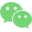微信公众号